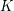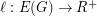# Georgakopoulos, Agelos

## End-Devouring Rays ★

Author(s): Georgakopoulos

Problem   Letbe a graph,a countable end of, andan infinite set of pairwise disjoint-rays in. Prove that there is a setof pairwise disjoint-rays that devourssuch that the set of starting vertices of rays inequals the set of starting vertices of rays in.

Keywords: end; ray

## Geodesic cycles and Tutte's Theorem ★★

Author(s): Georgakopoulos; Sprüssel

Problem   Ifis a-connected finite graph, is there an assignment of lengthsto the edges of, such that every-geodesic cycle is peripheral?

Keywords: cycle space; geodesic cycles; peripheral cycles

## Hamiltonian cycles in powers of infinite graphs ★★

Author(s): Georgakopoulos

Conjecture
\item Ifis a countable connected graph then its third power is hamiltonian. \item Ifis a 2-connected countable graph then its square is hamiltonian.

Keywords: hamiltonian; infinite graph

## Hamiltonian cycles in line graphs of infinite graphs ★★

Author(s): Georgakopoulos

Conjecture
\item Ifis a 4-edge-connected locally finite graph, then its line graph is hamiltonian. \item If the line graphof a locally finite graphis 4-connected, thenis hamiltonian.

Keywords: hamiltonian; infinite graph; line graphs